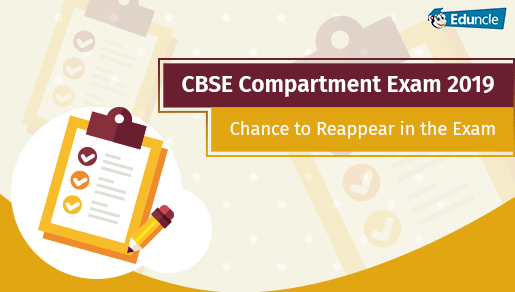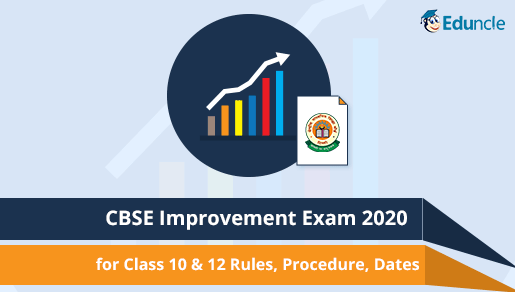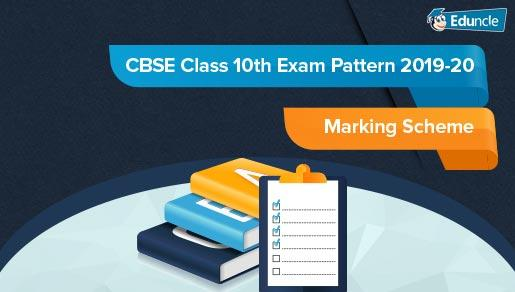Classes FollowShubham Gupta @Eduncle posted an Article
September 5, 2019 • 3:59 pm

# How to Study for CBSE Class 9 Maths 2019-20? Get the Complete Details!The CBSE Class 9 exams are the most critical as the students have to prepare themselves for the all India level competition in Class X & XII and the other competitive exams after the school.

Maths play a vital role in preparing a candidate for the further competition. As we all know that CBSE changed the exam curriculum and introduced the Board Exams after 8 long years. So, the class IX exam becomes very crucial for the students who are going to face tough competition next year.

The recent changes made in the exam structure of Class 9 will help the students habituate with the examination structure for the Class 10 Board. So, the students need to be more aware and alert about the changes in the Class 9 exam syllabus and pattern so that based on it, they can make good strategies to score well.

## CBSE Class 9 Maths Syllabus

The CBSE Class IX Maths Syllabus comprises of the different units which have different weightage. The list of topics in the Class IX Syllabus are as follows:

### Unit I: Number Systems

The following topics come under the number system chapter:

Real Numbers

### Unit II: Algebra

The topics which are under the algebra are as follows:

Polynomials

Linear Equations in Two Variables

### Unit III: Coordinate Geometry

The topics which come under the Coordinate Geometry are as follows:

Coordinate Geometry

### Unit IV: Geometry

The topics under this category are as given below:

Introduction to Euclid’s Geometry

Lines and Angles

Triangles

Area

Circles

Constructions

### Unit V: Mensuration

The topics consist of this are as follows:

Areas

Surface Areas and Volumes

### Unit VI: Statistics & Probability

The Statistics & Probability chapter gives you an initial insight of the concept:

Statistics

Probability

Most of the class IX syllabus is covered in the above-mentioned Units. These topics can be mastered by the practice.

The unit-wise coverage can be done by the teachers in the following way:

 Unit Name Chapter Name Periods Number Systems Real Numbers 18 Algebra Polynomials 23 Linear Equations in Two Variables 14 Coordinate Geometry Coordinate Geometry 6 Geometry Introduction to Euclid’s Geometry 6 Lines and Angles 13 Triangles 20 Quadrilaterals 10 Area 7 Circles 15 Constructions 10 Mensuration Areas 4 Surface Areas and Volumes 12 Statistics & Probability Statistics 13 Probability 9

## CBSE Class 9 Mathematics Exam Pattern/Marks Distribution

The new exam pattern and marking scheme for the CBSE Class IX Mathematics exam is as follows which comprises of 80 marks for the annual examination and 20 marks for the internal assessment.

Annual Examination: The 80 marks of the annual examination is distributed in the following pattern:

 Units Unit Name Marks I Number Systems 08 II Algebra 17 III Coordinate Geometry 04 IV Geometry 28 V Mensuration 13 VI Statistics & Probability 10 Total 80

### CBSE Class 9 Mathematics Question Paper format

The Maths question paper of class 9 format is based on the different levels of questions. A total of 30 questions are divided into the four sections, viz., A, B, C and D.

Theoretical Paper: 80 marks

Internal Assessment/ Project Work: 20 Marks

Marking Scheme: PDF

Question Paper Structure:

 Time Duration 3 Hours Maximum Marks 80 marks Total No. of Questions 40 Type of Questions Very Short Answer Objective Type – 1 mark Short Answer I – 2 marks Short Answer II – 3 marks Long Answer – 4 marks

The important details which you need to keep in mind about the annual examination are:

Students have to secure 33% marks out of 80 marks on the subject.

Marks and Grades will be awarded separately to each subject.

9-point grading will be same as followed by the Board in Class XII.

Schools will conduct IX Class examination for 80 marks which will cover 100% syllabus of the subject.

Internal Assessment: The 20 marks of the internal assessment are distributed in the following manner:

The students must secure minimum 33% marks out of the overall 20 marks in the internal assessment which consists of:

 S.No. Internal Assessment Activities Maximum no. of marks 1 Pen paper test Multiple Assessment (5+5) 10 marks 2. Portfolio 5 marks 3. Lab Practical (Lab activities to be done from the prescribed books) 5 marks

Grading System for the Scholastic Areas:

 Marks Range Grade 91-100 A1 81-90 A2 71-80 B1 61-70 B2 51-60 C1 41-50 C2 33-40 D 32 & below E (Failed)

## CBSE Class 9 Maths Books

The study material for the Class IX Maths comprises of the books which will be quite useful in the exam. The list of the prescribed books is as given below:

Mathematics-Textbook for class IX-NCERT Publication.

Guidelines for Mathematics Laboratory in Schools, class IX-CBSE Publication.

Laboratory Manual- Mathematics, secondary stage-NCERT Publication.

Mathematics exemplar problems for class IX-NCERT Publication.

### Class 9 Maths Question Papers

The previous year papers for the class IX maths give the idea about the paper pattern and the types of questions in the exam. Since a long period of time, the CBSE repeats the previous years’ questions every year. The exam papers will give you the genuine idea about the mindset of the examiner and level of the questions.

## Preparation and Study Tips for the Class 9 Maths

The preparation and the study tips for the class 9 maths will help a student to prepare for the exam in a proper and planned way so that they can score good marks in the Maths. Some of the tips which you need to follow are:

Prepare a notebook of important formulas and theorems so that you can revise them a day before the exam.

Practice is the key to score marks in the exam. Practice different types of questions for Maths.

Solve the CBSE practice papers and sample papers in one sitting within the time constraint.

Give more importance to the topics which have high weightage.

Solve the previous year exam papers which will increase your confidence and give you a pre-exam feel. It also helps to brush up all your concepts before the exam.

Don’t just read or mug up the topics but practice them. This is the most important thing which you need to keep in mind as reading maths does not help you. Instead, make a routine and give some time for practice.

Topics such as Geometry, Mensuration, Algebra have a lot of formulae and concepts base questions on which a lot of questions are asked.

The chapter wise tips and tricks for the class 9 maths are as follows:

1) Number System: This chapter has the number of the new concepts which you need to learn such as real numbers and their types, rational and irrational numbers. Though the topic is small but sometimes many students face problems due to the new concepts. So, learn the definitions very well for all the types of numbers.

2) Polynomials: This is one of the easiest topics in which you need to understand the definition and various types of polynomials along with their coefficients and degrees.

3) Coordinate Geometry: You only need to understand the Cartesian plane, the graph, and its applications. Practising the NCERT book is enough.

4) Linear Equations in Two Variables: It includes the solution and graphs of the linear equations in two variables. The practice of making graphs and equations is necessary.

5) Introduction to Euclid’s Geometry: This is one of the theoretical chapters in maths, which has some definitions, axioms, and postulates. You just can learn these and their basic applications.

6) Lines and Angles: Learn to solve the different types of questions based on the angles and various theorems.

7) Triangles: In this chapter, you must learn various properties and types of triangles. Applying them in the practice tests will make this chapter stronger.

8) Quadrilaterals: You can prepare for this chapters based on the types of quadrilateral and their properties.

9) Areas of Parallelograms and Triangles: In this chapter, learn the formulas and theorems for different types of triangles and parallelograms with their applications.

10) Circles: The various theorems and properties of circles are important in this chapter. You can only learn them with practice.

11) Constructions: This is the practical topic which can be learned by drawing and making of the different types of angles and triangles.

12) Heron’s Formula: The formula and its application should be learned in finding the area of triangles and quadrilaterals.

13) Surface Areas and Volumes: The application of how to calculate the areas and volumes of cubes, cuboids, spheres, cylinders, and cones.

14) Statistics: The easiest topic in maths in which you must learn the collection of data and their representation in different types graphs, histograms and how to find mean median and mode.

15) Probability: This chapter comprises of the 4-5 questions in the annual exam.

The above chapter wise tips and trick will help you in the preparation in a very planned way. This is the most effective way in which you can divide your whole syllabus and then prepare topic wise. This will be quite effective in the fast preparation of the topics.

Here, we come to the end of this article. We hope that this will help you with your preparation. If you have any query regarding this blog, you can ask them in the comment section below.

All the Best!!

You Might Also LikeShowing 1 comments out of 1
• Prabitha Prasannakumar
Nice